# Atoms and their structurePage 5

#### WATCH ALL SLIDES

Symbols

Find the

number of protons

number of neutrons

number of electrons

Atomic number

Mass Number

Name

Na

24

11

Slide 49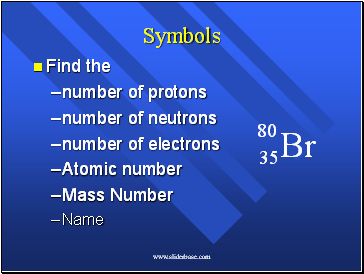Symbols

Find the

number of protons

number of neutrons

number of electrons

Atomic number

Mass Number

Name

Br

80

35

Slide 50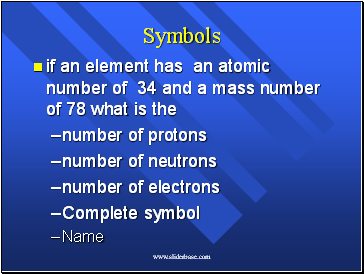Symbols

if an element has an atomic number of 34 and a mass number of 78 what is the

number of protons

number of neutrons

number of electrons

Complete symbol

Name

Slide 51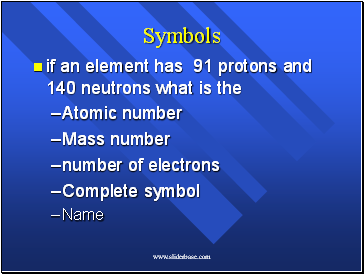Symbols

if an element has 91 protons and 140 neutrons what is the

Atomic number

Mass number

number of electrons

Complete symbol

Name

Slide 52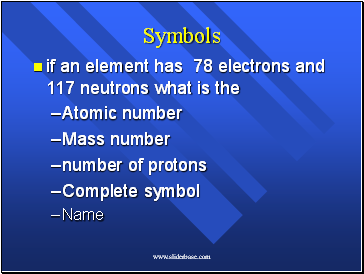Symbols

if an element has 78 electrons and 117 neutrons what is the

Atomic number

Mass number

number of protons

Complete symbol

Name

Slide 53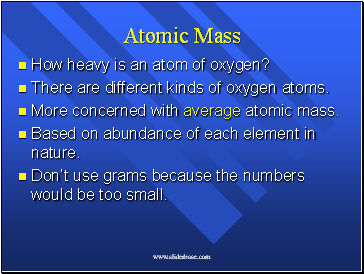## Atomic Mass

How heavy is an atom of oxygen?

There are different kinds of oxygen atoms.

More concerned with average atomic mass.

Based on abundance of each element in nature.

Don’t use grams because the numbers would be too small.

Slide 54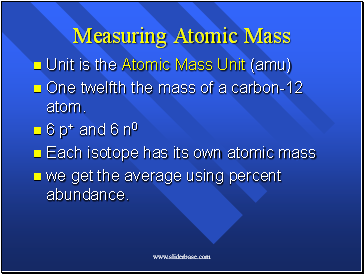Measuring Atomic Mass

Unit is the Atomic Mass Unit (amu)

One twelfth the mass of a carbon-12 atom.

6 p+ and 6 n0

Each isotope has its own atomic mass

we get the average using percent abundance.

Slide 55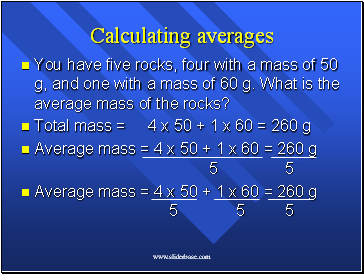## Calculating averages

You have five rocks, four with a mass of 50 g, and one with a mass of 60 g. What is the average mass of the rocks?

Total mass = 4 x 50 + 1 x 60 = 260 g

Average mass = 4 x 50 + 1 x 60 = 260 g 5 5

Average mass = 4 x 50 + 1 x 60 = 260 g 5 5 5

Slide 56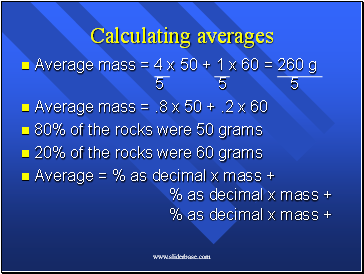Calculating averages

Average mass = 4 x 50 + 1 x 60 = 260 g 5 5 5

Average mass = .8 x 50 + .2 x 60

80% of the rocks were 50 grams

20% of the rocks were 60 grams

Average = % as decimal x mass + % as decimal x mass + % as decimal x mass +

Slide 57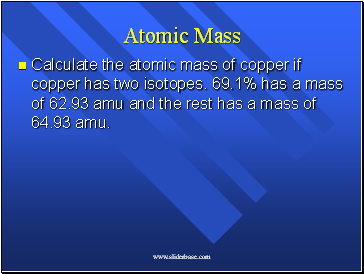Atomic Mass

Calculate the atomic mass of copper if copper has two isotopes. 69.1% has a mass of 62.93 amu and the rest has a mass of 64.93 amu.

Slide 58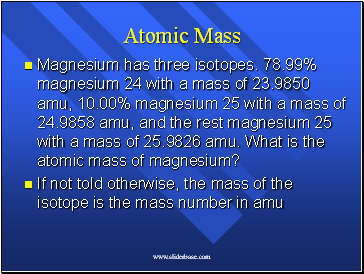Go to page:
1  2  3  4  5  6